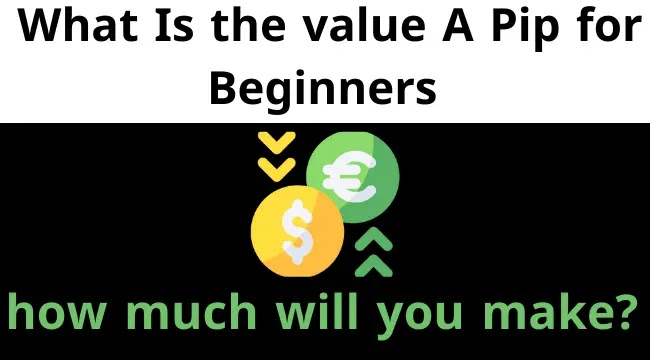recent
Hot news

# what is the pips in forex (Beginners Must Learn It at 2022)

Have you ever heard that the who controls the forex market pronounced a term called pip?

Surely you have heard this before. IF you want to know what it is, what it refers to, and how to trade forex. You should know what is the (pip) due to the importance that this term represents during your trades.### what is a pip in forex trading

PIP or pip is the unit of price action to see how much movement a particular pair has accomplished.

And to simplify further, you can understand the meaning of the point from the following example:

* Example

• the unit of measurement of distance is (meter).
• the unit of measurement of weight is (kilo)
• the unit of measurement of price movement is the pip (pip).

When we want to say that the price has moved, we say, for example, that the pair or price has moved, for example, 50 points or 50 PIP.

### how to calculate the value of a pip in forex

The point in the forex market can be calculated mathematically as 0.0001\$ that is, every 1000 pip is equal to 1 dollar, and in the same way, the point is equal to 1/100 of a cent, that is, every 100 points is equal to 1 cent.

*Examples

1. the euro-dollar rose from the price of 1.2000 to the price of 2010.

which means:-

• He has thus climbed by 10 points or 10 pips.

2. Eurodollar rose from the price of 1.2000 to the price of 1.2100.

which means:-

• It is thus increased by 100 points or 100 pips.

3. the pound/dollar pair fell from the price of 1.6050 to the price of 1.600.

which means:-

• It is thus reduced by 50 points or 50 pips.

### how to calculate pip value in Your Trading Account’s Currency?

The calculate pip value in forex can be calculated by dividing 1/10000 or 0.0001 by the exchange rate. The movement of a currency pair determines whether a trader has made a profit or loss from their positions at the end of the day.

#### how to calculate pip value when trading in an account in U.S. dollars?

The most traded currency pairs in the world include the U.S. dollar (USD). When the U.S. dollar is listed in second place in the pair, the PIP values are constant and do not change if you have a U.S. dollar-funded account.

The fixed point is:

• 10 USD for the standard contract, which is 100,000 PIP.
• 1 USD for a small contract, which is 10,000 PIP.
• 00.10 of USD for a small contract, which is 1,000 PIP.
• 0.100 of USD for a nano contract, which is 100 PIP.

These pip values apply to any pair in which the U.S. dollar is listed second, such as EUR / USD, GBP / USD, GBP / USD, AUD / USD.

#### Calculate pip value for a non-U.S. dollar account.

For example, a pair of USD/CAD. will have a fixed point value.

To find the Pip value when listing the Canadian dollar first, divide the fixed pip rate by the exchange rate.

For example, if the CAD / CHF exchange rate (CAD / CHF) is 0.70347, the pip.

It equals 1.42 Canadian dollars for a minor contract (1 Canadian dollar divided by 0.70347).

Perform this operation with any account currency to find the PIP values for the pairs that include that currency.

#### The Way To calculate pip value , While trading in other currency pairs

If your account currency is Euro and you want to know the Pip value of the AUD / CAD pair, remember that for someone with a CAD account, the standard will be 10 CAD for that pair. Convert that 10 CAD to euros by dividing it by the EUR/CAD rate.

A standard lot for someone with a Canadian dollar account would be 10 Canadian dollars.

Always keep in mind the currency that provides the Pip value is the second currency (YYY). When you know this, convert the fixed-point value in that currency to your currency by dividing it by XXX/YYY, and (XXX) is your account's currency.

If your account currency is in EUR and you want to calculate the value of a pip AUD/CAD, so to convert 10 Canadian dollars to euros, you can do it by dividing the euro rate by the Canadian dollar rate, If the price is 1.4612, the standard contract point value is EUR6.84.

The fixed Pip value in that currency to your own currency by dividing the Pip value by the pair (//YYY), and (XXX) is the currency of your own account, and.is the second currency.